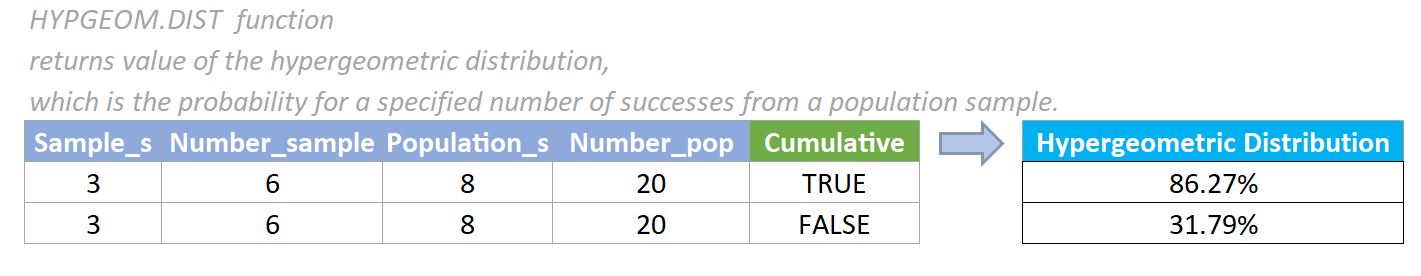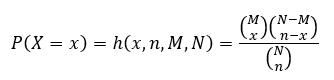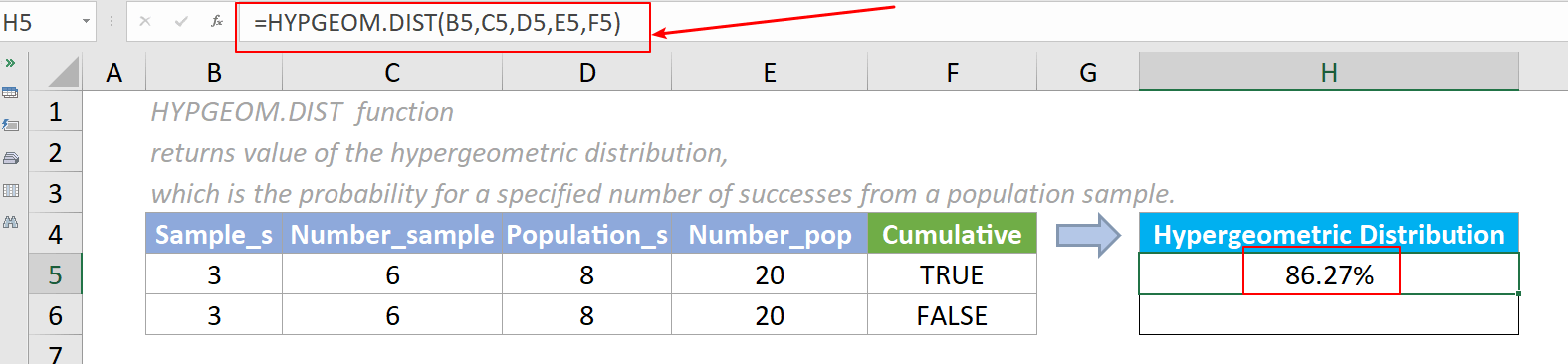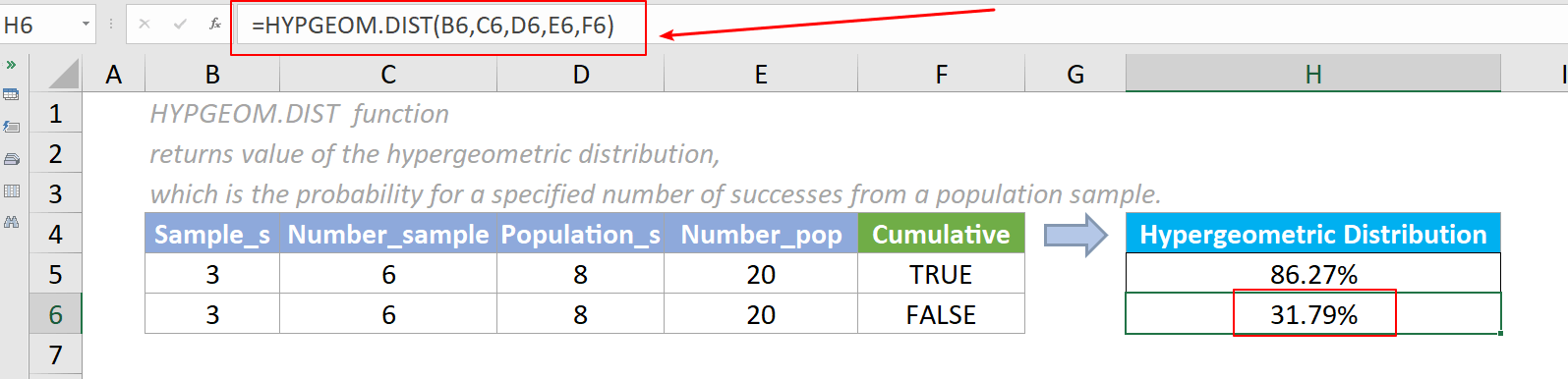## Excel HYPGEOM.DIST Function

The HYPGEOM.DIST function returns the value of the hypergeometric distribution, which is the probability for a specified number of successes from a population sample. The hypergeometric distribution is used to calculate probabilities of a given number of successes in a sample without replacement from a finite population.#### Syntax

=HYPGEOM.DIST (sample_s,number_sample,population_s,number_pop,cumulative)

#### Arguments

• Sample_s (required): The number of successes in the sample.
• Number_sample (required): The size of the sample.
• Population_s (required): The number of successes in the population.
• Number_pop (required): The size of the population.
• Cumulative (required): A logical value specifying the type of distribution to be calculated. It is either a TRUE or FALSE value.
If it is TRUE, HYPGEOM.DIST returns the cumulative distribution function, which calculates the probability of at most n successes from a specified number of trials.
If it is FALSE, HYPGEOM.DIST returns the probability density function, which calculates the probability of exactly n successes from a specified number of trials.

#### Return value

The HYPGEOM.DIST function returns a numeric value.

#### Function notes

1. The HYPGEOM.DIST function was newly introduced In Excel 2010, so it is unavailable in earlier versions.
2. The #VALUE! error value occurs if any of the supplied arguments is non-numeric.
3. The #NUM! error value occurs if any of the below situation occurs:
• The supplied sample_s is < 0;
• The supplied number_sample < 0 or > number_pop;
• The supplied population_s < 0 or > number_pop;
• The supplied number_pop ≤ 0.
4. Zero (0) will be returned when supplied sample_s is < (number_sample - number_population + population_s) value.
5. The equation for the hypergeometric distribution is:Where x means sample_s, n means number_sample, M means population_s, and N means number_pop.

#### Examples

##### Example One: Cumulative Distribution Function

In this case, you have a bag, inside which are 12 red balls and 8 blue balls. Now if you reach in the bag and pick 6 balls from it, what is the possibility of getting at most 3 blue balls?

Please copy the formula below into cell H5, then press the Enter key to get the result.

=HYPGEOM.DIST(B5,C5,D5,E5,F5)Note: We can also directly input values in the formula. The formula in cell H5 can be changed to:

=HYPGEOM.DIST(3,6,8,20,TRUE)

##### Example Two: Probability Density Function

Like the situation above, you also have a bag, inside which are 12 red balls and 8 blue balls. Now if you reach in the bag and pick 6 balls from it, what is the possibility of getting exactly 3 blue balls?

Please copy the formula below into cell H6, then press the Enter key to get the result.

=HYPGEOM.DIST(B6,C6,D6,E6,F6)Note: We can also directly input values in the formula. The formula in cell H6 can be changed to:

=HYPGEOM.DIST(3,6,8,20,FALSE)

#### Relative Functions:

• Excel EVEN Function
The EVEN function rounds numbers away from zero to the nearest even integer.

• Excel EXP Function
The EXP function returns the result of the constant e raised to the nth power.

### The Best Office Productivity Tools

#### Kutools for Excel - Helps You To Stand Out From Crowd

 Popular Features: Find, Highlight or Identify Duplicates  |  Delete Blank Rows  |  Combine Columns or Cells without Losing Data  |  Round without Formula ... Super VLookup: Multiple Criteria  |  Multiple Value  |  Across Multi-Sheets  |  Fuzzy Lookup... Adv. Drop-down List: Easy Drop Down List  |  Dependent Drop Down List  |  Multi-select Drop Down List... Column Manager: Add a Specific Number of Columns  |  Move Columns  |  Toggle Visibility Status of Hidden Columns  |  Compare Columns to Select Same & Different Cells ... Featured Features: Grid Focus  |  Design View  |  Big Formula Bar  |  Workbook & Sheet Manager | Resource Library (Auto Text)  |  Date Picker  |  Combine Worksheets  |  Encrypt/Decrypt Cells  |  Send Emails by List  |  Super Filter  |  Special Filter (filter bold/italic/strikethrough...) ... Top 15 Toolsets:  12 Text Tools (Add Text, Remove Characters ...)  |  50+ Chart Types (Gantt Chart ...)  |  40+ Practical Formulas (Calculate age based on birthday ...)  |  19 Insertion Tools (Insert QR Code, Insert Picture from Path ...)  |  12 Conversion Tools (Numbers to Words, Currency Conversion ...)  |  7 Merge & Split Tools (Advanced Combine Rows, Split Excel Cells ...)  |  ... and more

Kutools for Excel Boasts Over 300 Features, Ensuring That What You Need is Just A Click Away...#### Office Tab - Enable Tabbed Reading and Editing in Microsoft Office (include Excel)

• One second to switch between dozens of open documents!
• Reduce hundreds of mouse clicks for you every day, say goodbye to mouse hand.
• Increases your productivity by 50% when viewing and editing multiple documents.
• Brings Efficient Tabs to Office (include Excel), Just Like Chrome, Edge and Firefox.